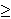## A Relaxation of the Hadwiger Conjecture

The famous Hadwiger Conjecture claims that every graph without Kn minor can be colored with fewer than n colors. The following result seems to be the first step in the direction towards the Hadwiger Conjecture:

Theorem 1 (Robertson and Seymour, unpublished): For every n there exists a polynomial-time algorithm (degree of whose polynomial time bound is independent of n) which finds, for every input graph G, either an (n-1)-coloring of G, or a minor of G that is not (n-1)-colorable.

At the Banff meeting on Structural and probabilistic approaches to graph coloring in September 2003, I proposed the following "strengthening" of Theorem 1: For every kn, there are only finitely many k-critical graphs that are Kn-minor-free. At the Danish Graph Theory conference in November 2003, Bjarne Toft observed that if we have one such graph, then we would have infinitely many by applying the Hajos operation. This shows that the conjecture is indeed equivalent to the Hadwiger Conjecture which implies that there are no such graphs. However, the following may be a weakening of Hadwiger's conjecture and a strengthening of Theorem 1:

Conjecture: For every kn, there are only finitely many 3-connected k-critical graphs that are Kn-minor-free.

Every 4-critical planar graph joined with the (n-5)-clique gives rise to an (n-1)-critical graph without Kn minor. This shows that neither Theorem 1 nor the above conjecture hold when the number of colors is further decreased.

References: